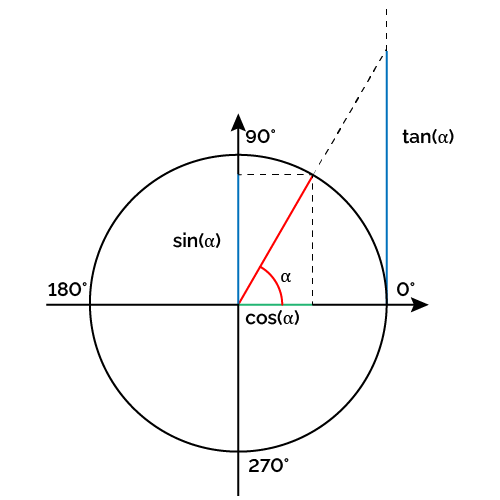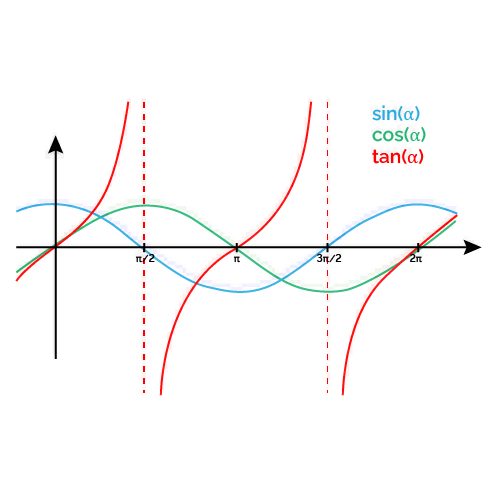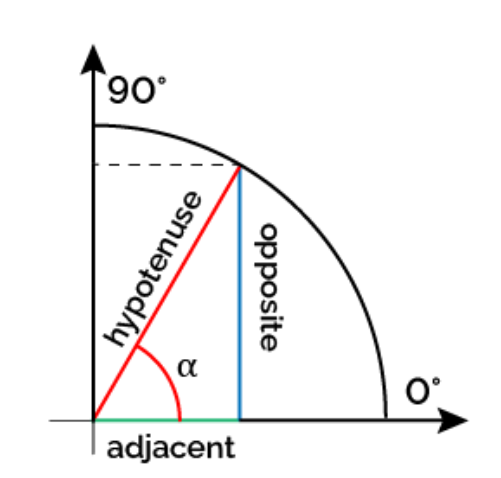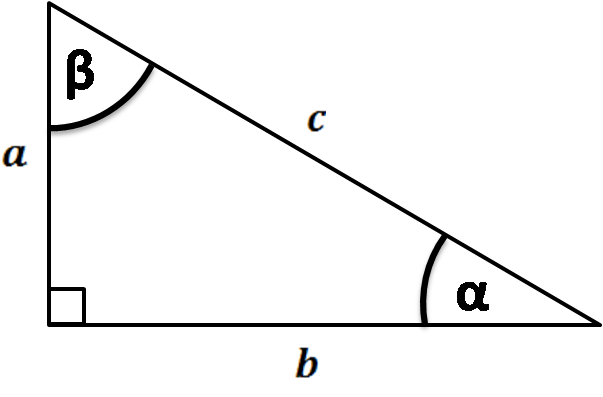# Sine Cosine Tangent Calculator

Created by Davide Borchia
Reviewed by Anna Szczepanek, PhD
Last updated: Jun 05, 2023

With our sine cosine tangent calculator, you will learn everything you need about the most important trigonometric functions: discover how to identify them, their relationship to right triangles, and how to calculate them in the blink of an eye!

## Trigonometric functions: how to calculate sine, cosine, and tangent

The trigonometric functions are mathematical functions that associate numerical values to angles according to specific rules. There are many trigonometric functions, but the most important are sine, cosine, and tangent. Since they take an angle as an argument, it's natural to identify them on a circle.

In the image below, you can see a trigonometric circle, a circle with radius $1$ where for a specific angle $\alpha$, we highlighted the segments corresponding to sine and cosine.Note how the tangent lies outside of the circle, tangent to it, as the name says. While sine and cosine are readily identifiable as the projections of the radius on the vertical and horizontal axis, we need to see the definition of the tangent to understand how to find it and visualize it:

$\tan(\alpha) = \frac{\sin(\alpha)}{\cos(\alpha)}$

In the picture below, we represented the three trigonometric functions: we calculated sine, cosine, and tangent values for the angles between $0\degree$ and $360\degree$.Even from this quick peek into trigonometry, you can identify many properties of these functions:

• The values calculated for sine, cosine, and tangent are periodic. In particular:
• Sine and cosine have periodicity equal to $360\degree$; and
• The tanget has periodicity $180\degree$.
• Sine and cosine have values comprised between $-1$ and $1$. The tangent assumes values between $-\infty$ and $\infty$.
• Sine and cosine switch values periodically in the four quadrants of the circle;
• Sine and cosine are "out of phase" by $90\degree$ with each other.

Keep exploring these functions to discover many other, almost endless, properties!

After this introduction to trigonometric functions, it's time to learn how to calculate sine, cosine, and tangent in a triangle. Jump to the next section!

## How do I calculate sine, cosine, and tangent in a triangle?

Right triangles and trigonometric functions share a deep connection: you can calculate the values of the latter and the geometric characteristic of the former swiftly without remembering any formulas: thanks to the neat graphic representation of the functions, you can always draw what you need on a piece of paper, and you'll recall the math in no time!

To understand the connection between right triangles and trigonometric functions, look at the detail of the previous image.We took an angle and highlighted the values of sine and cosine. As you can see, sine and cosine form a right angle; thus, they correspond to the catheti (legs) of the triangle. The radius of the circle becomes the hypotenuse. Immediately, you can identify the fundamental relationship between sine and cosine:

$\sin^2(\alpha)+\cos^2(\alpha) = 1,$

which we obtain from the Pythagorean theorem. But this alone would not tell us how to calculate sine, cosine, and tangent in a triangle. If you consider the angle $\alpha$, you can find the values of the functions thanks to a set of ratios between the lengths of the triangle's sides:

$\begin{split} \sin(\alpha) & = \frac{\mathrm{opposite}}{\mathrm{hypotenuse}}\\[1em] \cos(\alpha) & = \frac{\mathrm{adjacent}}{\mathrm{hypotenuse}}\\[1em] \tan(\alpha) & = \frac{\mathrm{opposite}}{\mathrm{adjacent}} \end{split}$

By rotating the triangle and placing the desired acute angle in the center of the trigonometric circle, you can calculate sine, cosine, and tangent for the second angle, too.

## Other Omni tools

Our sine, cosine, and tangent calculator allows you to see the values of the trigonometric functions directly for a specific angle. You can also insert the known information about a right triangle and see if they are enough to calculate the rest. If you found this tool useful, check out our other calculators:

## FAQ

### Why can I calculate sine, cosine, and tangent in a triangle?

Right triangles and trigonometric functions are deeply connected. If you look at sine and cosine on a trigonometric circle, you can see them forming a right angle, which allows you to define a right triangle, with catheti corresponding to sine and cosine.

By calculating the ratios between hypotenuse and catheti, you can find a measure of the "slope" of the segment in analysis, which is nothing but the result of a trigonometric function.

### How do I calculate sine, cosine, and tangent in a 3-4-5 triangle?

To calculate sine, cosine, and tangent in a 3-4-5 triangle, follow these easy steps:

1. Place the triangle in a trigonometric circle with an acute angle in the center.

2. Identify the adjacent and opposite catheti to the angle.

3. Compute the results of the trigonometric functions for that angle using the following formulas:

• sin(α) = opposite / hypotenuse;

• cos(α) = adjacent / hypotenuse; and

• tan(α) = opposite / adjacent.

4. Rotate and flip the triangle to find the values of sine, cosine, and tangent for the other acute angle, following the same steps.

### How do I calculate the sine in a right triangle?

To calculate the sine in a right triangle, follow these steps:

1. Identify the angle α in the analysis.

2. Assign the label opposite to the cathetus not touching the angle.

3. Assign the label adjacent to the cathetus touching the angle.

4. Calculate the sine with the ratio of the opposite cathetus and the hypotenuse:
sin(α) = opposite/hypotenuse.

5. To calculate the sine of the other acute angle ß, repeat Steps 2-4 or note that, in this case, sin(ß) = cos(α) thanks to the property sin(90° - α) = cos(α).

### How do I calculate the tangent if I know sine and cosine?

To calculate the tangent if you know sine and cosine, simply compute the ratio between these two quantities:

tan(α) = sin(α)/cos(α)

Since both sine and cosine go to zero in some instances (but never at the same time), the function has singularities where it is not defined: we find them at every argument of the form 90° + k × π, where k is an integer.

Davide Borchia
Trigonometric functions: sin, cos, tan...
Angle
deg
...or trigonometry in right triangle
Given
two sides
a
in
b
in
c
in
Angle α
deg
Angle β
degPeople also viewed…

### Involute function

Calculate the involute function in a single easy step using our involute function calculator.

### Obtuse triangle

Want to know whether your triangle is obtuse? Try the obtuse triangle calculator!

### Pizza size

This calculator will help you make the most delicious choice when ordering pizza.

### Secretary problem (Valentine's day)

Use the dating theory calculator to enhance your chances of picking the best lifetime partner.